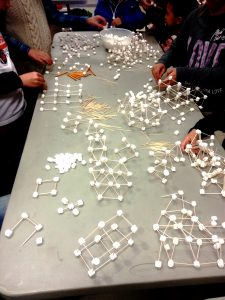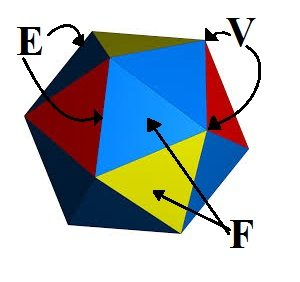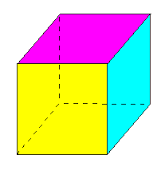# Marshmallow Geometry

## Marshmallow GeometryCombining Geometry, Balance of Equations, and the famous mathematician Euler for sweet architecture!

### Overview

Marshmallow Geometry is a classic, hands-on math engagement activity that invites free-play, exploration, and creativity in conjunction with studying geometry and the concept of "Balance of Equations". Building 3-dimensional structures with marshmallows also offers instant feedback in the builder's success, allowing the student to immediately learn from mistakes and apply that new knowledge to the task at hand.

Best of all for this activity, students are playing with marshmallows - who wouldn't pass up that sweet opportunity!

### Materials

• Marshmallows! Select 1-2 bags of the smallest size.
• Toothpicks (approximately 1 box per 1 bag of marshmallows)
• Helpful: a bowl to pour the marshmallows in (or multiple bowls if you have 5+ students)
• Optional: Medium sized marshmallows

### Project Details: 1 - Exploring Shapes and GeometryEspecially for younger students who are just beginning to learn about shapes, set aside some time to just play and explore with creating all kinds of shapes and learning about their properties.

Explore polygons, which are 2-dimensional shapes: Triangles, Squares, Pentagons, Hexagons, Heptagons, Octogons, etc. Grab some toothpicks and marshmallows and make an n-sided figure. Once your shape is closed, count how many sides you have. What shape is it?

Explore polyhedra, which are 3-dimensional shapes (singular: polyhedron): Cubes, Tetrahedrons, Square Pyramids, Rectangular Prisms, Triangular Prisms, Dodecahedrons, etc.

Get creative creating your own shapes and structures!

### Project Details: 2 - Balance of Equations

What does Balance of Equations mean, and what does it have to do with marshmallows?Balance of Equations is exactly what it sounds like: the value on both sides of an equal sign must be equal to each other, like a scale balancing.

In school, we grow to think of the equal sign to mean "gives us an answer of".

For example, what's 2+3 = ? Did you say "5"?
That's true, adding 2 and 3 does give us an answer of 5, but is 5 the only solution that balances that equation?
Of course not! What about 4+1? 7-2? 20/4? -50+55? All of these answers are creative ways of balancing the equation. The equal sign is not meant to say "just write the answer here", but we often forget that.

Students exploring Marshmallow Geometry can discover for themselves how to balance equations by exploring the amazing formula discovered by mathematician Leonhard Euler ("oil-er").If we define the following:

V = the number of Vertexes: the points or corners of an object,
F = the number of Faces: the flat surfaces of an object,
E = the number of Edges: the straight lines that connect the points or corners of an object,

then, for any non-self-intersecting polyhedron (a closed, 3-dimensional shape made up of flat edges [no curves], points, and faces), all polyhedra (plural of polyhedron) will follow this rule:

V + F = E + 2For example, a cube has 8 corners, 6 faces, and 12 edges. 8 + 6 = 12 + 2 (14 = 14).

In the world of marshmallows and toothpicks, we re-define the variables to be:

M = the number of Marshmallows, or vertexes
F = the number of Faces
T = the number of Toothpicks, or edges

And so we have:

M + F = T + 2

Do you believe Euler? Put the formula to the test! Start grabbing some toothpicks and marshmallows, and create all kinds of 3-D structures! When you're done, count the the number of marshmallows (vertexes), the number of faces, and the number of toothpicks (edges), and see if your equation is balanced!

### Discussion

Our brains better retain information when we engage both our hands and minds in the activity. Now that we've explained how to engage the hand, what are some discussion questions to engage the mind during the activity?

1. How many different ways can you make a triangle with marshmallows and toothpicks? Do triangles always have to have sides that are equal lengths? Can you make examples of triangles with non-equal sides?

2. Compare all of the different triangles that you made. How do the angles compare? Which triangle has angles that are all the same size? Is it possible to make a triangle with two obtuse (larger than 90˚) angles and only one acute (less than 90˚) angle?

3. Make a square and then make a rectangle with your marshmallows and toothpicks. What do these shapes have in common? What is their difference?

4. How many ways can you make a pentagon with marshmallows and toothpicks? Do pentagons always have to have sides that are equal lengths? Can you make examples of pentagons with non-equal sides?

5. Make a complete square. Now pull out the end of one toothpick from a marshmallow. Is it still a square? Is it still a polygon? Why or why not?

6. When building 3-D structures, which shapes help you build a stronger structure? Which shapes are not as strong? Why do you think this is?

### Supporting Resources

MathIsFun.com is rich in clear, thorough explanations of mathematical concepts. Math Engaged recommends this site for both introducing new concepts and reinforcing already-learned concepts for students and parents alike. Click to find wonderfully explained concepts in geometry: http://www.mathsisfun.com/geometry/index.html
See how many of these topics from MathIsFun.com you can explore by playing with marshmallows and toothpicks!

MathIsFun.com's page on Polyhedra also discusses Euler's formula: https://www.mathsisfun.com/geometry/polyhedron.html

And you can read MathIsFun.com's page solely about Euler's formula here: https://www.mathsisfun.com/geometry/eulers-formula.html

Looking for a great team-building exercise that involves marshmallows? Try the Great Marshmallow Challenge! This activity has been a Math Engaged favorite at our Creative Math Camps over the years. Learn more about this great activity: http://www.tomwujec.com/design-projects/marshmallow-challenge/

## Did you find this activity helpful?

Math Engaged is committed to empowering students of all ages to take learning into their own hands and explore mathematics with an open mind. We strive to provide materials, resources, and engaging learning opportunities at no cost to families as much as possible.

If you found this activity helpful, please let us know - if we know that families enjoy these activities, we'll work even harder to get more up faster at MathEngaged.org.